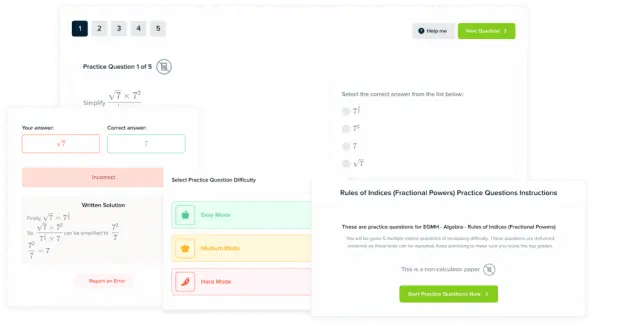# Unit Conversions

GCSEAQA

## Unit Conversions

In an exam question, you may be asked to give your answer in a different unit than you normally use. To do this, you need to know how to convert units

## SI Units

SI units are the standard units we use to measure quantities. The main SI units are shown in the table below.

 Quantity Unit Symbol time second $\text{s}$ length metre $\text{m}$ mass kilogram $\text{kg}$ electric current amp $\text{A}$ temperature kelvin $\text{K}$ amount of a substance mole $\text{mol}$ luminous intensity candela $\text{cd}$

All other units can be made by combining SI units. You can work out which SI units make up another unit using the equation for that quantity.

Example: Express $1 \text{ N}$ (newton) in SI units.

[2 marks]

The newton is the unit of force and the equation we use to calculate force is $\bold{F=ma}$

The unit of mass is the kilogram $\text{(kg)}$

The unit of acceleration is metres per square second $\text{(m/s}^2\text{)}$

Because mass and acceleration are multiplied together in the equation, you multiply these units together.

$\text{ N}=\textcolor{00d865}{\text{kg}} \times \textcolor{10a6f3}{\text{m/s}^2}=\text{kgm/s}^2$

Therefore,

$\bold{1} \textbf{ N} \bold{=1} \textbf{ kgm/s} \bold{^2}$

GCSEAQA

## Unit Prefixes

Prefixes are used to avoid writing large numbers or standard form. You should be aware of the following prefixes and their meanings:

 Prefix Symbol Standard Form Number giga G $\times 10^9$ $\times 1\, 000 \, 000 \, 000$ mega M $\times 10^6$ $\times 1 \, 000 \, 000$ kilo k $\times 10^3$ $\times 1 \, 000$ milli m $\times 10^{-3}$ $\times 0.001$ micro $\mu$ $\times 10^{-6}$ $\times 0.000\, 001$ nano n $\times 10^{-9}$ $\times 0.000 \, 000\, 001$

For example:

• $1 \text{ GW}= 10^9 \text{ W} = 1 \, 000 \, 000 \, 000 \text{ W}$
• $5 \, \mu \text{m} = 5 \times 10^{-6} \text{ m} = 0.000 \, 005 \text{ m}$.
• $2.5 \text{ kg} = 2.5\times 10^3 \text{ g} = 2500 \text{ g}$
• $500 \text{ nm} = 500 \times 10^{-9} \text{ m} = 5 \times 10^{-7} \text{ m} = 0.000 \, 000 \, 7 \text{ m}$

You should also know the meaning of the following words:

 Name Number Standard Form Number of Zeros Thousand $1 \, 000$ $1 \times 10^3$ $3$ Million $1 \, 000 \, 000$ $1 \times 10^6$ $6$ Billion $1\, 000 \, 000 \, 000$ $1 \times 10^9$ $9$
GCSEAQA

## Common Conversions

There are some common conversions you should also know.

Miles$\bold{\rightarrow}$Kilometres

There are $1.61 \text{ km}$ in every mile. This means we can use the following equations to convert between kilometres and miles:

$\text{km}= \text{miles}\times 1.61 \, \, \, \, \, \, \text{ miles}=\dfrac{\text{km}}{1.61}$

• $\text{km}=$ the number of kilometres
• $\text{miles}=$ the number of miles

Degrees Celsius$\bold{\rightarrow}$Kelvin

Absolute zero, $0\text{ K}$ is equal to $-273 \degree \text{C}$. This means we can use the following equations to convert between degrees Celsius and kelvin.

$\text{K}=\degree \text{C} + 273 \, \, \, \, \, \, \degree \text{C}=\text{K} - 273$

• $\text{K}=$ the temperature in kelvin $\text{(K)}$
• $\degree \text{C}=$ the temperature in degrees Celsius $(\degree \text{C})$

Tonne$\bold{\rightarrow}$Kilogram

$1$ tonne is equal to $1000$ kilograms. You therefore use the following equations to convert between tonnes and kilograms:

$\text{kg}=\text{tonnes} \times 1000 \, \, \, \, \, \, \text{tonnes}=\dfrac{\text{kg}}{1000}$

• $\text{kg}=$ the number of kilograms
• $\text{tonnes}=$ the number of tonnes
GCSEAQA

## Unit Conversions Example Questions

Current units – Amps $\text{(A)}$

Time units – Seconds $\text{(s)}$

Charge units – $\text{A}\times \text{s} = As$

So $\bold{1} \textbf{ C} = \bold{1} \textbf{ As}$Gold Standard Education

$\text{Mega} = 1 \, 000 \, 000 = 10^6$

So in $2$ megabytes there are:
$\bold{2 \, 000 \, 000}$ or $\bold{2 \times 10^6}$ or $\bold{2} \textbf{ million}$ bytesGold Standard Education

\begin{aligned} \text{kelvin} &= \degree \text{C} + 273 \\ &= 21 + 273 \\ &= \bold{294} \textbf{ K} \end{aligned}Gold Standard EducationProduct